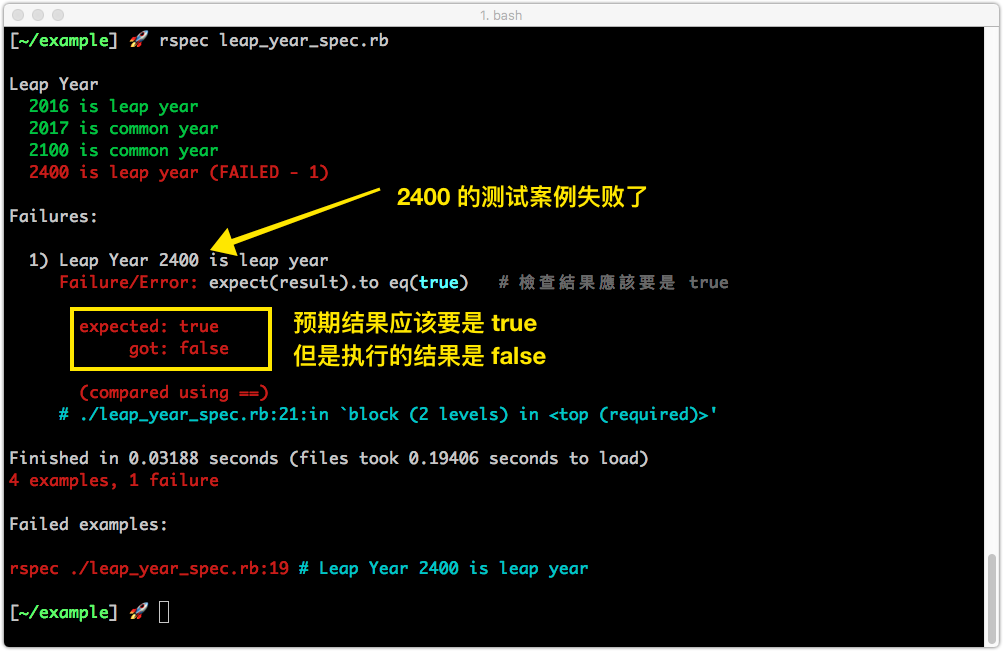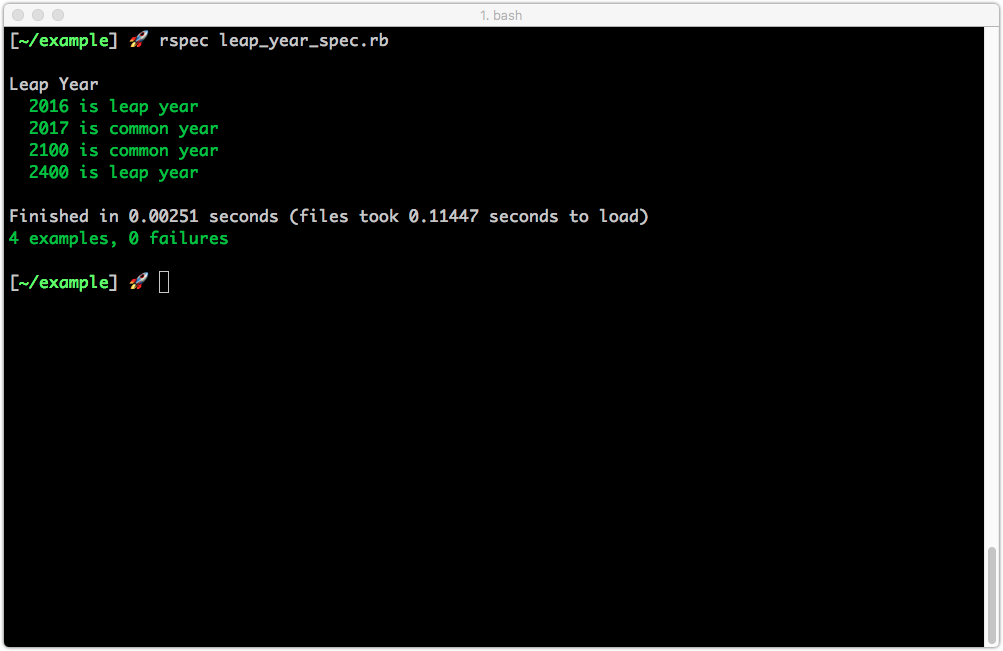# Rails 自動化測試 課程簡介

• 閏年程序
• 停車計費應用
• Web API 自動化測試

# 一個簡單的閏年程序

## 1-1 閏年程序(第一版)

• 西元年分除以400可整除，為閏年。
• 西元年分除以4可整除但除以100不可整除，為閏年。
• 西元年分除以4不可整除，為平年。
• 西元年分除以100可整除但除以400不可整除，為平年

``````def is_leap_year?(year)
if year % 4 == 0
return true
else
return false
end

# 同學們可能會發現這不是正確的版本，讓我們繼續做下去
end
``````

``````require_relative './leap_year'
is_leap_year?(2016)   # => 應該得到 true
is_leap_year?(2017)   # => 應該得到 false

``````

``````is_leap_year?(2100)   # => 得到 true，但是應該要 false 才對

``````

## 1-2 閏年程序(第二版)

``````def is_leap_year?(year)
if year % 4 == 0
if year % 100 == 0
return false
else
return true
end
else
return false
end
end
``````

``````require_relative './leap_year'
is_leap_year?(2016)   # => 應該得到 true
is_leap_year?(2017)   # => 應該得到 false
is_leap_year?(2100)   # => 應該得到 false 了
``````

## 1-3 使用 RSpec 自動化測試

`gem install rspec`

``````--format documentation
--color
``````

``````require_relative './leap_year'

describe "Leap Year" do
it "2016 is leap year" do
result = is_leap_year?(2016)  # 把 2016 傳進去
expect(result).to eq(true)    # 檢查結果應該要是 true
end

it "2017 is common year" do
result = is_leap_year?(2017)  # 把 2017 傳進去
expect(result).to eq(false)   # 檢查結果應該要是 false
end

it "2100 is common year" do
result = is_leap_year?(2100)  # 把 2100 傳進去
expect(result).to eq(false)   # 檢查結果應該要是 false
end

it "2400 is leap year" do
result = is_leap_year?(2400)  # 把 2400 傳進去
expect(result).to eq(true)    # 檢查結果應該要是 true
end

end

``````### 1-4 閏年程序(完成版)

``````def is_leap_year?(year)
if year % 4 == 0
if year % 100 == 0
if year % 400 == 0
return true
else
return false
end
else
return true
end
else
return false
end
end

``````## 1-5 重構閏年程序(最終版)

``````def is_leap_year?(y)
y % 400 == 0 || ( y % 4 == 0 && y % 100 != 0)
end

``````